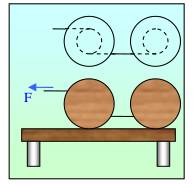# Two reels rolling without slipping

bznm

## Homework Statement[/B]
I have two cylindric reels laying on the ground. They are identical: mass M, composed by a cylinder of radius R and two side cylinders of radius 2R. I have to find angular acceleration and work done by F in delta t.

2. The attempt at a solution

On the first cylinder the torque is ##\tau_1=3RF-RT##, while on the second is ##\tau_2=RT##
as the rope isn't extensible the two cylinders should have the same angular acceleration.
So ##\alpha_1=\alpha_2##
##3RF-3RT=RT##
##T=-\frac{3}{2} F##

##\alpha=R/I *3/2 F=\frac{3RF}{2I}##
Angular velocity of cylinder is ##\omega = \alpha t = \frac{3RF}{2I} t##.
Kinetic energy of the first cylinder is ##1/2 I \omega^2= \frac{9R^2F^2t^2}{8I}## and this is also the work done by F plus the work done by T on the first cylinder. But as ##T/F=-3/2## and they are applied in the same way with respect to the displacement I expect ##W_T/W_F=-3/2## so ##W_F=2/5 \Delta K##. Is it correct?
Thanks a lot

Homework Helper
On the first cylinder the torque is ##\tau_1=3RF−RT##, while on the second is ##\tau_2=RT##
as the rope isn't extensible the two cylinders should have the same angular acceleration.
Interesting, can you explain ?
The rope can roll up (or off) on the reels, I presume ?
 A bit late, but I get it. Let me sit back and think a little longer before postingbznm
Interesting, can you explain ?
The rope can roll up (or off) on the reels, I presume ?
Oh my gosh, you're right.. I'm totally wrong(i don't know why but I considered it as a rod) and I don't know what to do now :/

Homework Helper
But the "##\tau_1=3RF−RT##, while on the second is ##\tau_2=RT##" is correct. That gives angular acceleration and therefore also (uniformly) accelerated translation

bznm
But the "##\tau_1=3RF−RT##, while on the second is ##\tau_2=RT##" is correct. That gives angular acceleration and therefore also (uniformly) accelerated translation
I tried: (where A is friction force)
## F-A-T=M a_1 = M \alpha_1 2R ##
## T-A=M a_2 = M \alpha_2 2R ##

Subtracting, I get: ##F-2T=M(2R)(\alpha_1-\alpha_2)##
##F-2T=M(2R)\frac{3RF-2RT}{I}##
##T=F\frac{1+6MR^2 / I}{2+4MR^2 / I}##

Is it correct?
What about the work? Is it correct my reasoning in applying kinetic energy theorem?